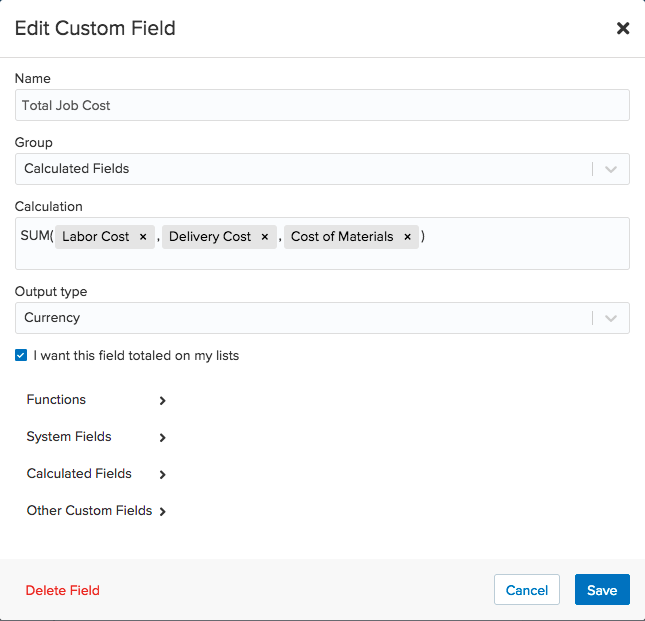# Calculated Fields

Calculated fields allow you to have the data in your custom fields calculated from data hosted in other fields. Calculated fields currently support system and custom fields that are numeric, currency, and date fields.

### Creating Calculated Fields

Any Admin on your account can create a calculated field by navigating to the Custom Fields section of Account Settings. Simply click to add a new field for the desired record type and select Calculated Field from the dropdown. From there you will give the field a name and select a custom field group if you desire.

Next, you will select the calculated function. You can sum, subtract, multiply, and divide values, or choose from several of our date functions. Then you will choose the fields you would like to calculate, you can choose from system fields as well as custom fields. You will need to enter a comma to separate each value and close the parentheses at the end. Finally, you will choose the output value.Once the custom field is saved it will automatically calculate the values that are already entered. You can return to your saved list, add the newly created column, and see the calculated field in action!

### Function Types

• Sum: The total amount of the fields selected.
• Subtract: Finds the difference between your selected fields.
• Multiply: Multiplies the values of the selected fields.
• Divide: Divides the first value selected by the second.
• Datediff: (start_date, end_date, unit): Select a start date and an end date, then enter the unit wrapped in quotations. The supported units are day, week, and month. The value shown will be the number of units between the start and end date.
• Day (Date): This function will calculate the numeric day of the month for your selected date field.
• Weekday (Date): This function will calculate the numeric day of the week for your selected date field.
• Month (Date): This function will calculate the numeric month of the year for your selected date field.
• Year (Date): This function will calculate the year for your selected date field.
• EDate (Date, number_of_months): This function will take the date shown in the selected field, and add the chosen number of months. This could be useful for tracking scheduled outreach.
• WeekNum (date): This function will calculate the numeric week of the year for your selected date field. (i.e. 1st or 10th week of the year)